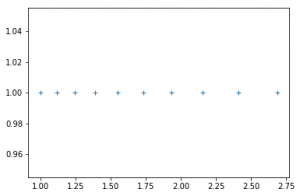# numpy.geomspace() in Python

`numpy.geomspace() `is used to return numbers spaced evenly on a log scale (a geometric progression).
This is similar to `numpy.logspace()` but with endpoints specified directly. Each output sample is a constant multiple of the previous.

Syntax : numpy.geomspace(start, stop, num=50, endpoint=True, dtype=None)

Parameters :
start : [scalar] The starting value of the sequence.
stop : [scalar] The final value of the sequence, unless endpoint is False. In that case, num + 1 values are spaced over the interval in log-space, of which all but the last (a sequence of length num) are returned.
num : [integer, optional] Number of samples to generate. Default is 50.
endpoint : [boolean, optional] If true, stop is the last sample. Otherwise, it is not included. Default is True.
dtype : [dtype] The type of the output array. If dtype is not given, infer the data type from the other input arguments.

Return :
samples : [ndarray] num samples, equally spaced on a log scale.

Code #1 : Working

 `# Python3 Program demonstrate ` `# numpy.geomspace() function ` ` `  `import` `numpy as geek ` ` `  ` `  `print``(``"B\n"``, geek.geomspace(``2.0``, ``3.0``, num ``=` `5``), ``"\n"``) ` ` `  `# To evaluate sin() in long range  ` `point ``=` `geek.geomspace(``1``, ``2``, ``10``) ` `print``(``"A\n"``, geek.sin(point)) `

Output :

```B
[ 2.          2.21336384  2.44948974  2.71080601  3.        ]

A
[ 0.84147098  0.88198596  0.91939085  0.95206619  0.9780296   0.9948976
0.99986214  0.98969411  0.96079161  0.90929743]
```

Code #2 : Graphical Representation of numpy.geomspace()

 `# Graphical Represenation of numpy.geomspace() ` `import` `numpy as geek  ` `import` `pylab as p  ` `%` `matplotlib inline   ` ` `  `# Start = 1  ` `# End = 3  ` ` `  `# Samples to generate = 10  ` `x1 ``=` `geek.geomspace(``1``, ``3``, ``10``, endpoint ``=` `False``)  ` `y1 ``=` `geek.ones(``10``)  ` `   `  `p.plot(x1, y1, ``'+'``)  `

Output :My Personal Notes arrow_drop_upCheck out this Author's contributed articles.

If you like GeeksforGeeks and would like to contribute, you can also write an article using contribute.geeksforgeeks.org or mail your article to contribute@geeksforgeeks.org. See your article appearing on the GeeksforGeeks main page and help other Geeks.

Please Improve this article if you find anything incorrect by clicking on the "Improve Article" button below.

Article Tags :

Be the First to upvote.

Please write to us at contribute@geeksforgeeks.org to report any issue with the above content.